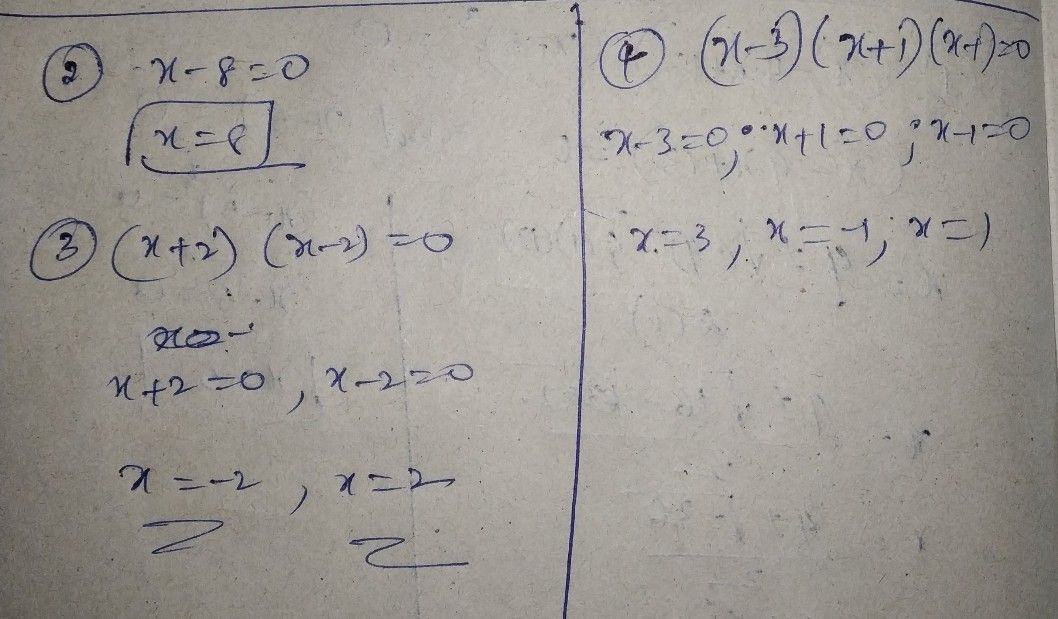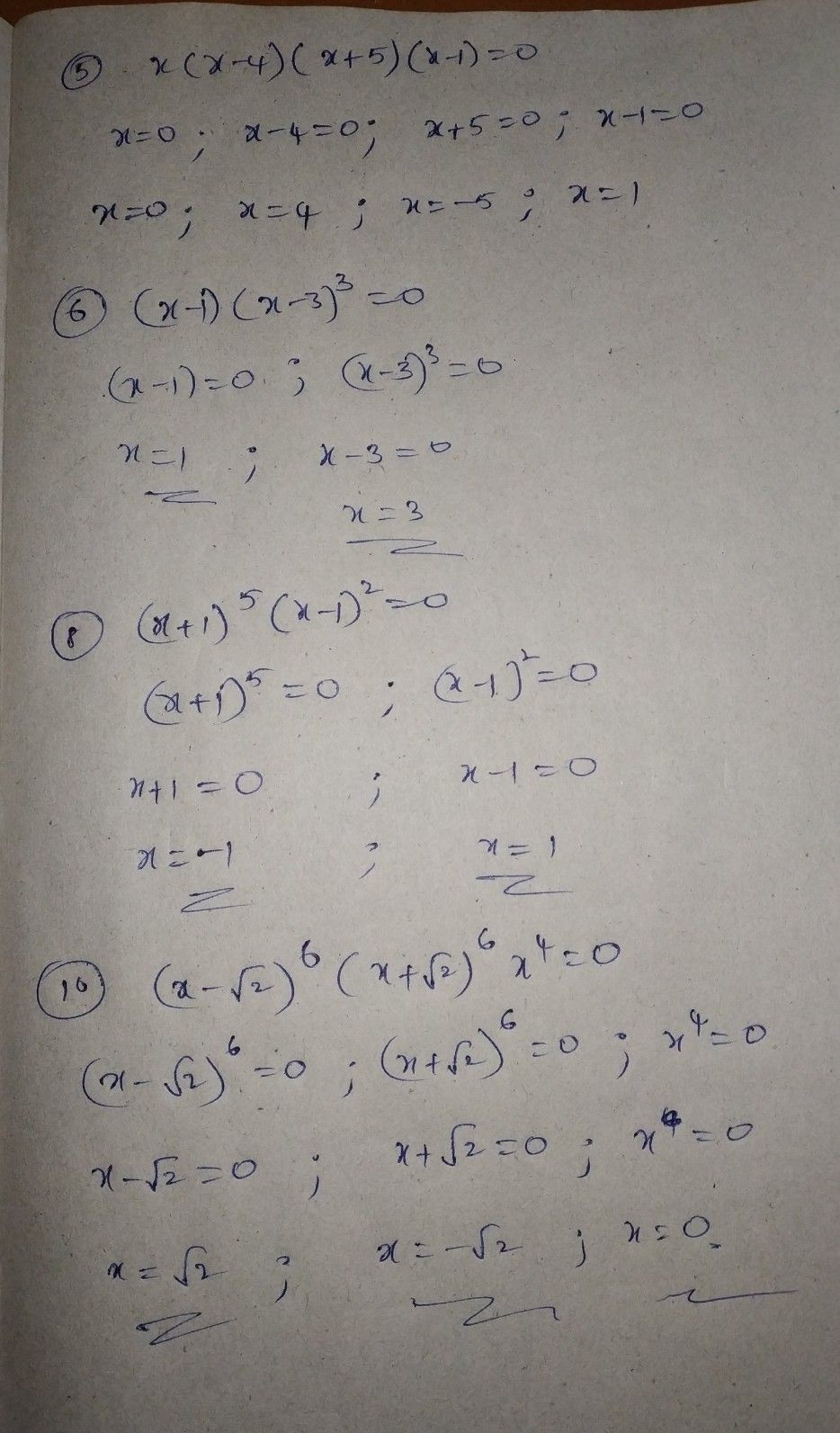Symbol
ProblemActivity $2=$ Finding the Number of Roots of Polynomial Equations Some polynomial equations are given below. Complete the table and answer the questions that follow. (If a root occurs twice, count it twice; if thrice, count it three times, and so on. The first 'one is done for you) Polynomial Equation Degree Real Roots of Number of an Equation Real Roots $1$ $\left(x+1\right)^{2}\left(x-5\right)=0$ $-1\left(2$ times); 5 3 $2.$ $x-8=0$ $3$ $\left(x+2\right)\left(x-2\right)=0$ $4$ $\left(x-3\right)\left(x+1\right)\left(x-1\right)=0$ $5$ $x\left(x-4\right)\left(x+5\right)\left(x-1\right)=0$ $6$ $\left(x-1\right)\left(x-3\right)^{3}=0$ $7.$ $\left(x^{2}-4x+13\right)\left(x-5\right)^{3}=0$ $8.$ $\left(x+1\right)^{5}\left(x-1\right)^{2}=0$ $9.\left(x^{2}+4\right)\left(x-3\right)^{3}=0$ $10.\left(x-\sqrt{2} \right)^{6}\left(x+\sqrt{2} \right)^{6}x^{4}=0$
Other
Search count: 129
Question content
Solution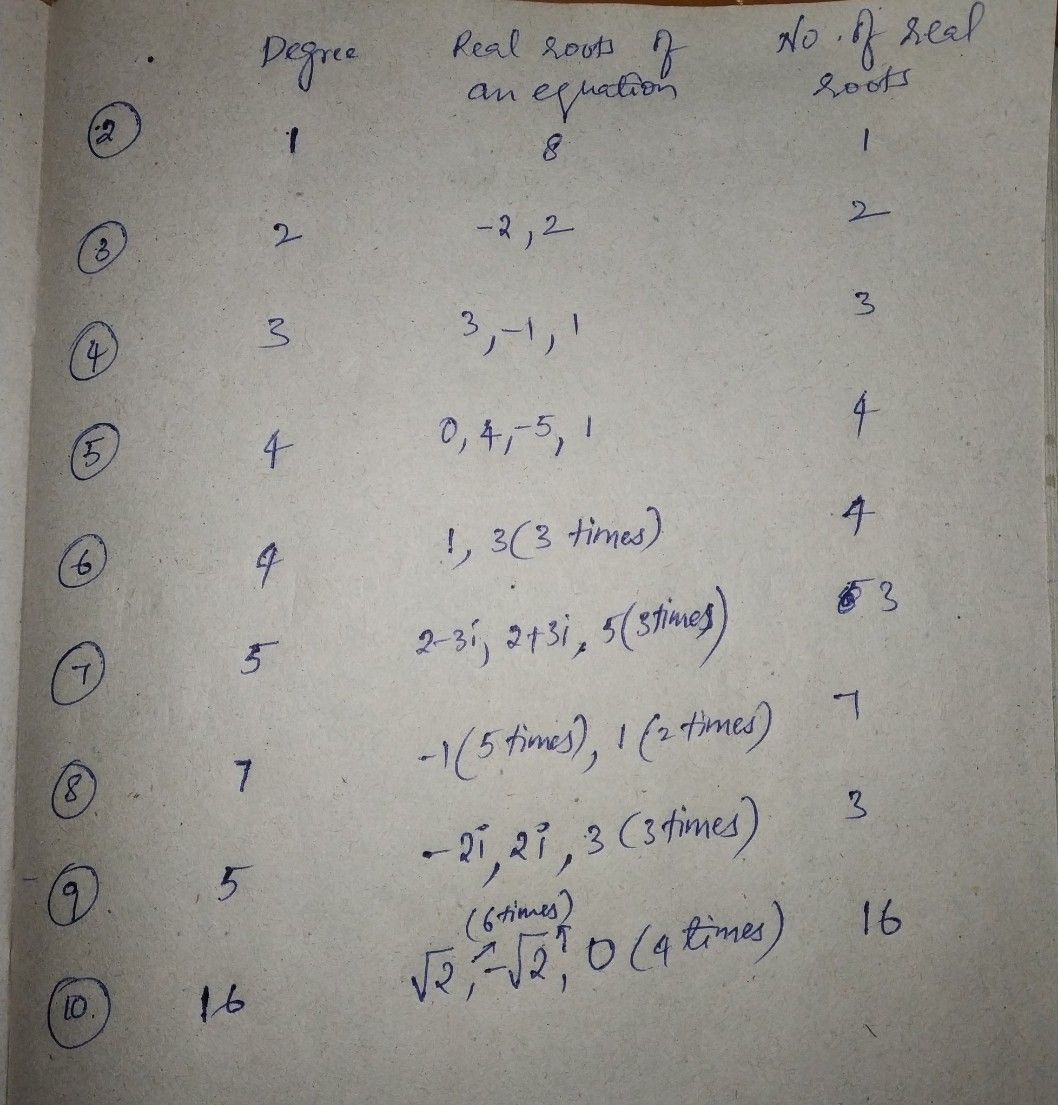Student
thank u ma'am can u send the solution on how did u solve thatexcept for 7th and 9th everything is similar just equate them to the zeroStudent
ok ma'am noted
ma'am where's the solution :)
or computation of that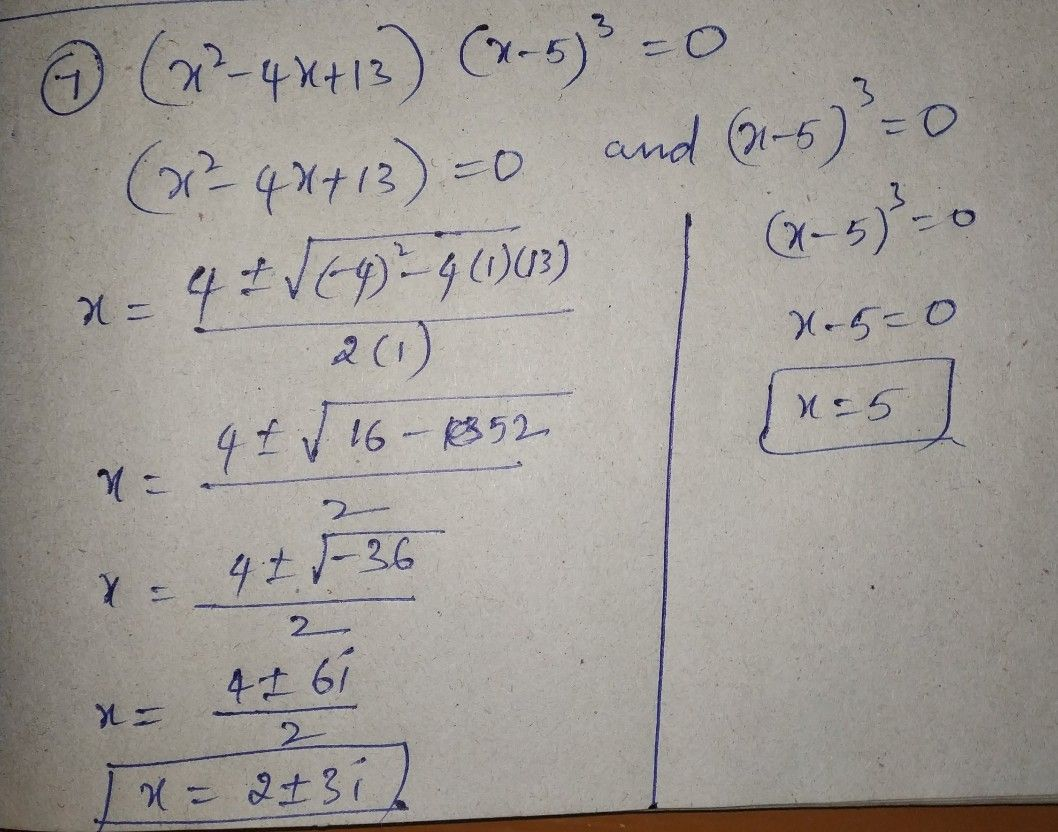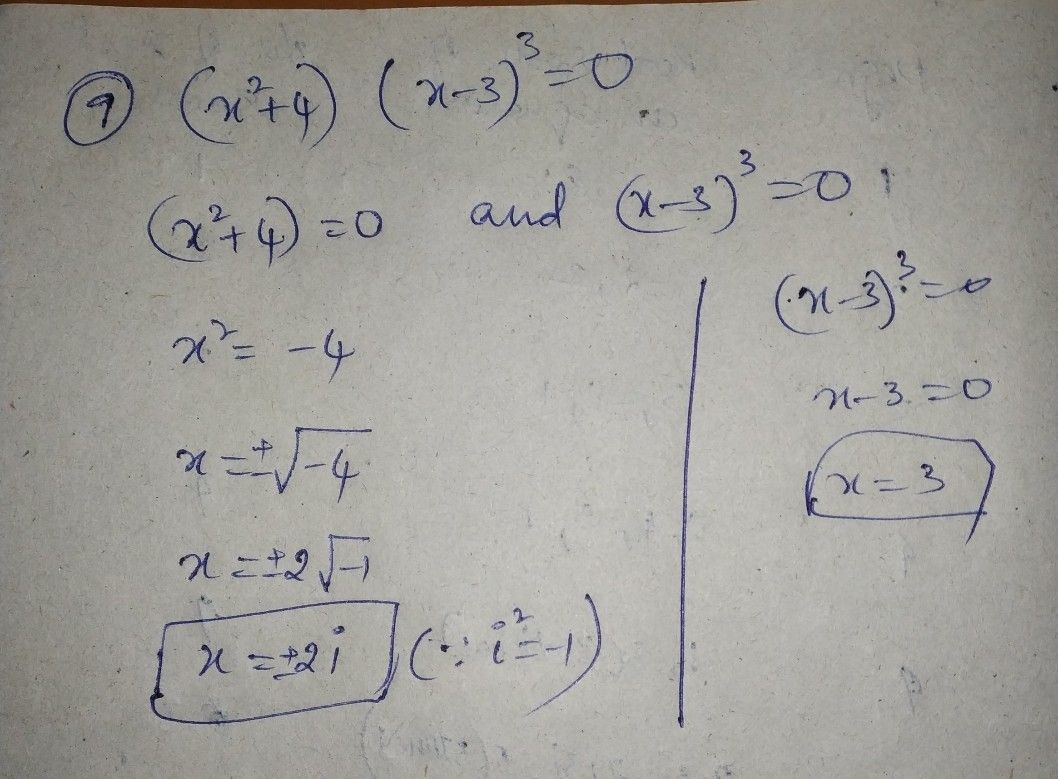did u understand how to do the remainingStudent
no ma'am but thank you i really appreciated itjust equate them to the zero like in the above examples
most welcomeStudent
yes ma'am thank you i will?❤️
ma'am I'm just wondering of what is the solution/computation of 2,3,4,5,6,8,10